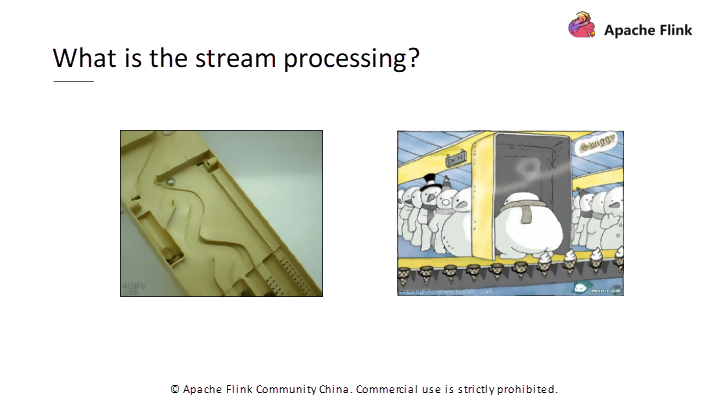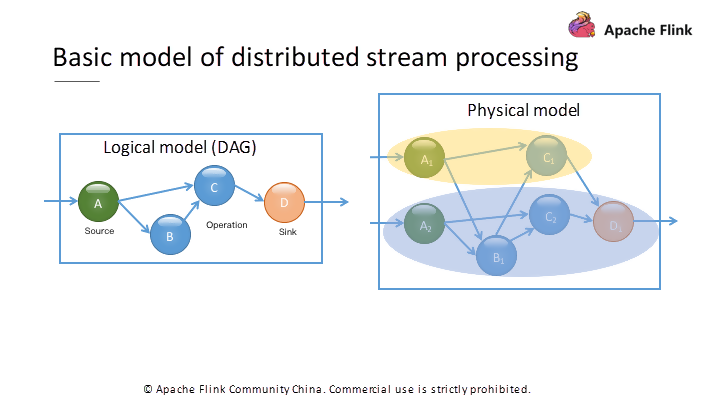# Basic Concepts of Stream ProcessingFigure 1. On the left is a coin classifier.Figure 2. A DAG computing logic graph and an actual runtime physical model.
`TopologyBuilder builder = new TopologyBuilder();builder.setSpout("spout", new RandomSentenceSpout(), 5);builder.setBolt("split", new SplitSentence(), 8).shuffleGrouping("spout");builder.setBolt("count", new WordCount(), 12).fieldsGrouping("split", new Fields("word"));`
`StreamExecutionEnvironment env = StreamExecutionEnvironment.getExecutionEnvironment();DataStream<String> text = env.readTextFile ("input");DataStream<Tuple2<String, Integer>> counts = text.flatMap(new Tokenizer()).keyBy(0).sum(1);counts.writeAsText("output");`

`// 1. Set the runtime environmentStreamExecutionEnvironment env = StreamExecutionEnvironment.getExecutionEnvironment();// 2. Configure the data source to read dataDataStream<String> text = env.readTextFile ("input");// 3. Perform a series of transformationsDataStream<Tuple2<String, Integer>> counts = text.flatMap(new Tokenizer()).keyBy(0).sum(1);// 4. Configure the Sink to write data outcounts.writeAsText("output");// 5. Submit for executionenv.execute("Streaming WordCount");`

`public class GroupedProcessingTimeWindowSample {    private static class DataSource extends RichParallelSourceFunction<Tuple2<String, Integer>> {        private volatile boolean isRunning = true;        @Override        public void run(SourceContext<Tuple2<String, Integer>> ctx) throws Exception {            Random random = new Random();            while (isRunning) {                Thread.sleep((getRuntimeContext().getIndexOfThisSubtask() + 1) * 1000 * 5);                String key = "类别" + (char) ('A' + random.nextInt(3));                int value = random.nextInt(10) + 1;                System.out.println(String.format("Emits\t(%s, %d)", key, value));                ctx.collect(new Tuple2<>(key, value));            }        }        @Override        public void cancel() {            isRunning = false;        }    }    public static void main(String[] args) throws Exception {        StreamExecutionEnvironment env = StreamExecutionEnvironment.getExecutionEnvironment();        env.setParallelism(2);        DataStream<Tuple2<String, Integer>> ds = env.addSource(new DataSource());        KeyedStream<Tuple2<String, Integer>, Tuple> keyedStream = ds.keyBy(0);        keyedStream.sum(1).keyBy(new KeySelector<Tuple2<String, Integer>, Object>() {            @Override            public Object getKey(Tuple2<String, Integer> stringIntegerTuple2) throws Exception {                return "";            }        }).fold(new HashMap<String, Integer>(), new FoldFunction<Tuple2<String, Integer>, HashMap<String, Integer>>() {            @Override            public HashMap<String, Integer> fold(HashMap<String, Integer> accumulator, Tuple2<String, Integer> value) throws Exception {                accumulator.put(value.f0, value.f1);                return accumulator;            }        }).addSink(new SinkFunction<HashMap<String, Integer>>() {            @Override            public void invoke(HashMap<String, Integer> value, Context context) throws Exception {                  // 每个类型的商品成交量                  System.out.println(value);                  // 商品成交总量                                  System.out.println(value.values().stream().mapToInt(v -> v).sum());            }        });        env.execute();    }}`